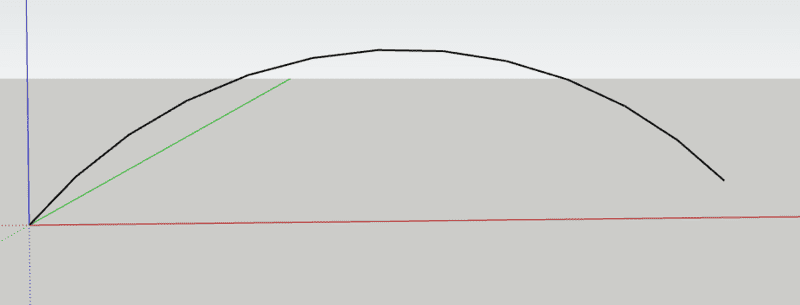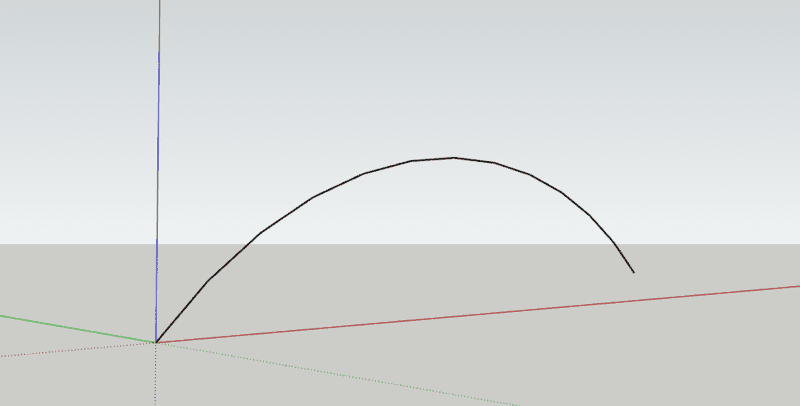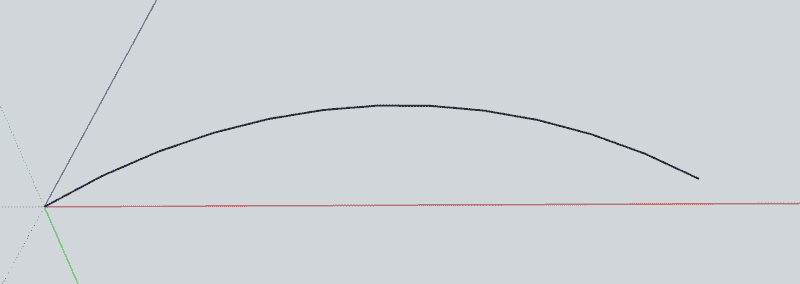# Projection of 3D Plane from a certain perspective on the 2D Plane

• A
• jisbon

#### jisbon

TL;DR Summary
Given a 3D Plane, assuming I have a trajectory motion in the XYZ plane and have some coordinates of the projection at every 1 second, how do I project this motion on a person that is standing at another point in the 3D plane in a 2D format?
Hello all!
As seen in the summary, I'm not sure if anyone can understand, but I will try to make this as clear as possible.
Working in the 3D Plane:
Given that there is a trajectory motion in the 3D Plane, and I have the coordinates of the motion at every 1s interval.
This means at t=1s, the coordinates could be at (… ,..., …) etc. and it will form a invert-u shape like a normal trajectory motion as shown below.Now, assuming I have a person looking at this motion. This person can be standing anywhere in the 3D Plane. Hence, to his perspective, this trajectory motion will definitely look different from different locations. This can be seen below:(The same projectile motion, but at a different location)

Furthermore, the looks of the motion will also change once we take into account of the height difference. This means that the person could be at the bottom staring at the motion, resulting in a different shape as shown.(Again, the same motion, but looking under it this time aka an elevation angle is incurred)

Given that I have the location of the person, the location of the projectile motion at some intervals, how am I able to 'sketch'/'create' the graph that is projected onto the person given that the user has the locations (as previously stated), the elevation angle etc.?

I have thought of calculating the distance at each interval of the projectile motion to the person, but am stuck there afterwards, not knowing how do I create/sketch the perspective of the motion to the person. Any help will be greatly appreciated.

Thank you!